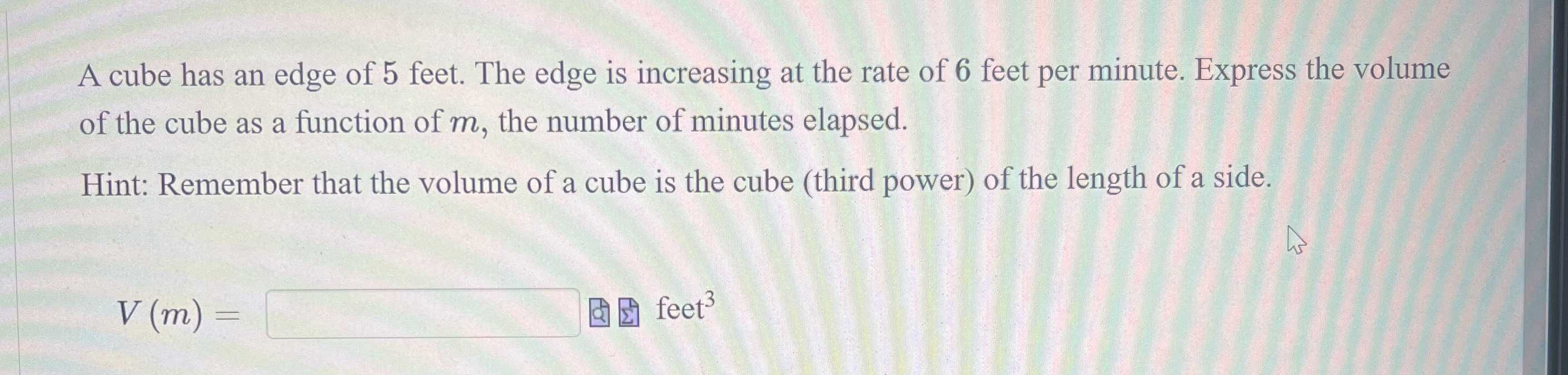### ¿Todavía tienes preguntas de matemáticas?

Pregunte a nuestros tutores expertos
Algebra
PreguntaA cube has an edge of $$5$$ feet. The edge is increasing at the rate of $$6$$ feet per minute. Express the volume of the cube as a function of $$m ,$$ the number of minutes elapsed.

Hint: Remember that the volume of a cube is the cube (third power) of the length of a side.

$$V ( m ) = \square$$ feet $$^ { 3 }$$

$$V = (5+ 6m)^3$$